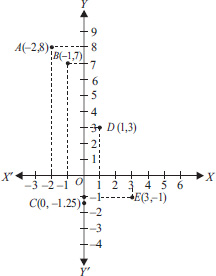Coordinate Geometry (Mathematics) Class 9 - NCERT Questions
Q 1.

How will you describe the position of a table lamp on your study table to another person?

SOLUTION:

To describe the position of a table lamp placed on the table, let us consider the table lamp as P and the table as a plane.
Now choose two perpendicular edges of the table as the axes OX and OY. Measure the perpendicular distance ‘a’ cm of P (lamp) from OY. Measure the perpendicular distance ‘b’ cm of P (lamp) from OX.
Thus, the position of the table lamp P is described by the ordered pair (a, b).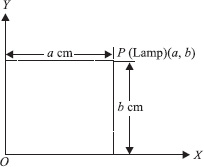Q 2.

(Street Plan): A city has two main roads which cross each other at the centre of the city. These two roads
are along the North-South direction and East-West direction.
All other streets of the city run parallel to these roads and are 200 m apart. There are 5 streets in each direction.
Using 1 cm = 200 m, draw a model of the city on your notebook. Represent the road/ street by single line.
There are many cross streets in your model. A particular cross street is made by two streets, one running in the North-South
direction and another in the East-West direction. Each cross street is referred to in the following manner. If the 2nd
street is running in the North-South direction and 5th in the East-West direction meet at some crossing, then we will call
this cross-street (2, 5). Using this convention, find
(i) how many cross streets can be referred to as (4, 3)?
(ii) how many cross streets can be referred to as (3, 4)?

SOLUTION:

(i) A unique cross street as shown by the point A(4, 3).
(ii) A unique cross street as shown by the point B(3, 4).
The two cross streets are uniquely found because of the two reference lines we have used for locating them.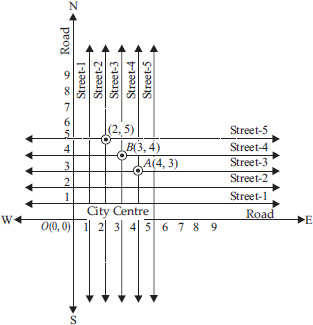Q 3.

Write the answer of each of the following questions :
(i) What is the name of horizontal and the vertical lines drawn to determine the position of any point in the Cartesian plane?
(ii) What is the name of each part of the plane formed by these two lines?
(iii) Write the name of the point where these two lines intersect.

SOLUTION:

(i) The horizontal line: x-axis and the vertical line: y-axis.
(ii) Each part is called “Quadrant”.
(iii) Origin

Q 4.

See the given figure and write the following :
(i) The coordinates of B.
(ii) The coordinates of C.
(iii) The point identified by the coordinates (–3, –5).
(iv) The point identified by the coordinates (2, –4)
(v) The abscissa of the point D.
(vi) The ordinate of the point H.
(vii) The coordinates of the point L.
(viii) The coordinates of the point M.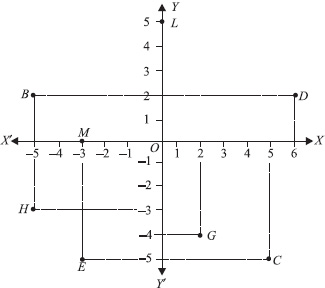SOLUTION:

From the figure, we have
(i) The coordinates of B are (–5, 2).
(ii) The coordinates of C are (5, –5).
(iii) The point E is identified by the coordinates (–3, –5).
(iv) The point G is identified by the coordinates (2, – 4).
(v) The abscissa of the point D is 6.
(vi) The ordinate of the point H is –3.
(vii) The coordinates of the point L are (0, 5).
(viii) The coordinates of the point M are (–3, 0).

Q 5.

In which quadrant or on which axis do each of the points (–2, 4),(3, –1), (–1, 0),(1, 2) and
(–3, –5) lie? Verify your answer by locating them on the Cartesian plane.

SOLUTION:

In the point (–2, 4), the abscissa is negative and the ordinate is positive.
(–, +) belongs to 2nd quadrant.
(–2, 4) lies in the second quadrant.
The point (3, –1) is having positive abscissa and negative ordinate.
(3, –1) lies in the 4th quadrant.
The point (–1, 0) is having negative abscissa and zero ordinate.
The point (–1, 0) lies on the negative x-axis.
The point (1, 2) is having the abscissa as well as ordinate positive.
Point (1, 2) lies in the 1st quadrant.
The point (–3, –5) is having the abscissa as well as ordinate negative.
Point (–3, –5) lies in the 3rd quadrant.
These points are plotted in the Cartesian plane as shown in the following figure as A(–2, 4); B(3, –1); C(–1, 0); D(1, 2) and E (–3, –5).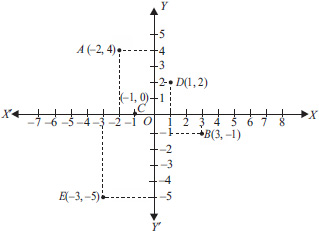Q 6.

Plot the points (x, y) given in the following table on the plane, choosing suitable units of distance on the axes.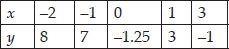SOLUTION:

The given points are (–2, 8), (–1, 7), (0, –1.25), (1, 3) and (3, –1). To plot these points:
(i) We draw X′OX and YOY′ as axes.
(ii) We choose suitable units of distance on the axes.
(iii) To plot (–2, 8), we start from O, take (–2) units on x-axis and then (+8) units on y-axis. We mark the point as A (–2, 8).
(iv) To plot (–1, 7), we start from O; take (–1) units on x-axis and then (+7) units on the y-axis. We mark the point as B(–1, 7).
(v) To plot (0, –1.25), we move along 1.25 units below the x-axis on the y-axis and mark the point as C(0, –1.25).
(vi) To plot (1, 3), we take (+1) unit on the x-axis and then (+3) units on the y-axis. We mark the point as D(1, 3).
(vii) To plot (3, –1), we take (+3) units on the x-axis and then (–1) unit on the y-axis. We mark the point E(3, –1).